# Monte Carlo simulation¶

Using the probability distribution the probability distribution of a random vector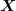, we seek to evaluate the following probability: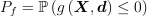Here,is a random vector,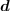a deterministic vector,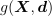the function known as limit state function which enables the definition of the event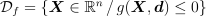.

If we have the set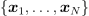of N independent samples of the random vector, we can estimate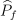as follows: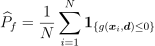where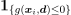describes the indicator function equal to 1 if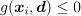and equal to 0 otherwise; the idea here is in fact to estimate the required probability by the proportion of cases, among the N samples of, for which the event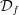occurs.

By the law of large numbers, we know that this estimation converges to the required value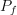as the sample size N tends to infinity.

The Central Limit Theorem allows one to build an asymptotic confidence interval using the normal limit distribution as follows: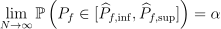with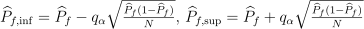and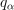is the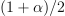-quantile of the standard normal distribution.

One can also use a convergence indicator that is independent of the confidence level $alpha$: the coefficient of variation, which is the ratio between the asymptotic standard deviation of the estimate and its mean value. It is a relative measure of dispersion given by: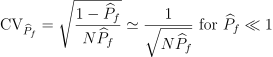It must be emphasized that these results are asymptotic and as such needs that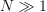. The convergence to the standard normal distribution is dominated by the skewness ofdivided by the sample size N, it means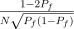. In the usual case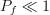, the distribution is highly skewed and this term is approximately equal to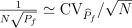. A rule of thumb is that if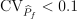with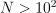, the asymptotic nature of the Central Limit Theorem is not problematic.

The method is also referred to as Direct sampling, Crude Monte Carlo method, Classical Monte Carlo integration.